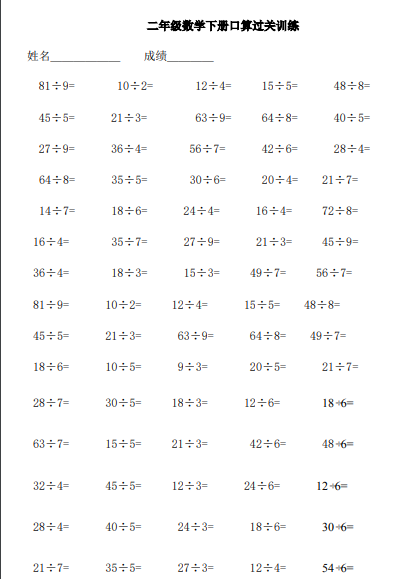81÷9= 10÷2= 12÷4= 15÷5= 48÷8=
45÷5= 21÷3= 63÷9= 64÷8= 40÷5=
27÷9= 36÷4= 56÷7= 42÷6= 28÷4=
64÷8= 35÷5= 30÷6= 20÷4= 21÷7=
14÷7= 18÷6= 24÷4= 16÷4= 72÷8=
16÷4= 35÷7= 27÷9= 21÷3= 45÷9=